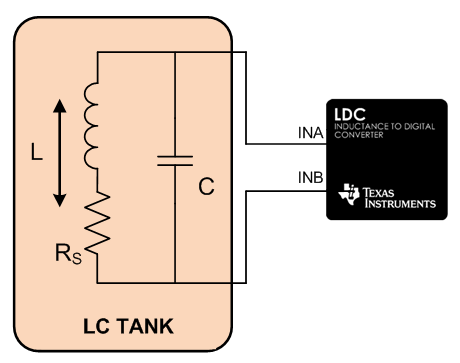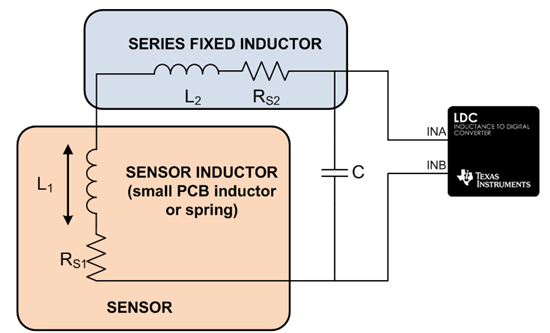# Inductive sensing: How to use a tiny 2mm PCB inductor as a sensor

Recently, a customer asked me if he could use a tiny PCB coil as the sensing element for the LDC1000 inductance-to-digital converter (LDC). The PCB coil had only three turns per PCB layer on a four-layer board and measured 2mm in diameter. By itself, the inductance of the PCB coil was too low to produce an LC tank, which oscillates with the LDC1000. Since the location of the sensor was highly space constrained, I recommended adding a fixed series inductor to solve his dilemma.

Inductance-to-digital converters use an external LC tank circuit as a sensing element, which is comprised of an inductor with a series parasitic resistance and a parallel capacitor, as shown in Figure 1.Figure 1: The LC tank senses proximity of conductive targets

The range of tank oscillation frequency is limited by the drive strength of the inductance-to-digital converter output driver. To ensure stable oscillation of the LC tank, the LDC1000 requires a sensor oscillation frequency between 5kHz and 5MHz, while ensuring that the equivalent parallel resistance (RP) at resonance remains between 798Ω and 3.98MΩ. These boundary conditions can create scenarios where the impedance of the chosen sensor is insufficient to design a suitable LC tank oscillator.

When I measured the three-turn, four-layer PCB inductor with a network analyzer, I found that the inductance at the maximum allowed an oscillation frequency of 5MHz is 150nH. At this frequency, I measured a series resistance of 0.54Ω.

The LC tank oscillation frequency is given by:

Therefore, a 6.8nF capacitor would be required to reduce the tank oscillation frequency to 5MHz. However, the equation for the equivalent parallel resistance at resonance:

shows that RP is only 40.8Ω, which is significantly less than the minimum 798Ω that the LDC1000 tank driver requires to ensure stable oscillation. No capacitor value can be added to the 150nH PCB coil to produce an LC tank that operates within the fsensor and RP boundary conditions of the LDC1000.

To overcome this, I added a fixed series inductor to the sensor inductor, as shown in Figure 2. The series inductor increases the sensor impedance without operating at an unsupported oscillation frequency. Suitable fixed series inductors include surface mount (SMD) inductors and multi-layer PCB coils. The series inductor should not act as a second sensor, so it is important to either physically isolate it from movable conductive materials in its proximity or use a shielded SMD inductor.

Note that resolution is impacted when you use a fixed series inductor, because only a portion of the combined inductance acts as a sensor (a good analogy is adding a DC offset to an AC signal). Therefore, you should keep the series inductor as small as possible while comfortably satisfying the boundary conditions of the LDC1000. This will produce the best possible resolution.Figure 2: Adding a series inductor increases sensor impedance

I added a TDK MLZ1608E4R7M multi-layer inductor in an 0603 package, which has a nominal inductance of 4.7μH. The sensor inductor and the series fixed inductor showed a combined inductance measurement of 5.3μH at 5MHz and a combined series resistance of 6.4Ω.

To allow some margin, I added a 270pF tank capacitor to produce a 4.2MHz oscillation frequency. The equivalent parallel resistance at resonance of this LC tank is 3.1kΩ, well within the specified RP range of the LDC1000.

If your LDC design is space constrained and dictates use of a physically small sensor coil that has low inductance, or if you’re using a small spring as the sensing element, all you need to do is add a fixed series inductor. This will provide LC tank oscillation within the LDC boundary conditions.

Have a question about designing with an inductance-to-digital converter? Search for answers and get help in our Inductive Sensing forum.